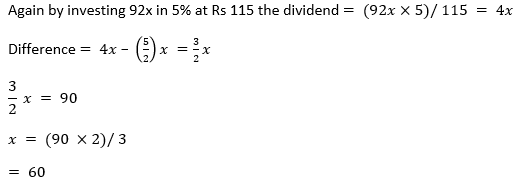# ML Aggarwal Solutions Class 10 Maths Chapter 3 Shares and Dividends

1. Find the dividends received on 60 shares of Rs 20 each if 9% dividend is declared.
Solution:
Given,
Nominal value (N.V) of one shares = Rs.20
∴ value of 60 shares = Rs.20 × 60
= Rs.1200
Given that rate of dividend = 9%
∴ total dividend = Rs.1200 × 9%
= 1200 × (9/100)
= Rs 108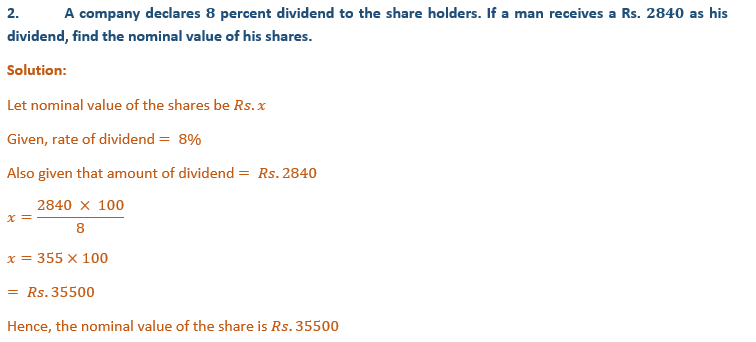3. A man buys 200 ten-rupee shares at Rs.12.50 each and receives a dividend of 8%. Find the amount invested by him and the dividend received by him in cash.
Solution:
Given nominal value of one shares = Rs.10
Number of shares = 200
∴ face value of 200 shares = 10 × 200
= Rs.2000
Now, the amount invested for the purchase of 200 shares at the rate of Rs.12.50 each
= 12.50 × 200
= Rs.2500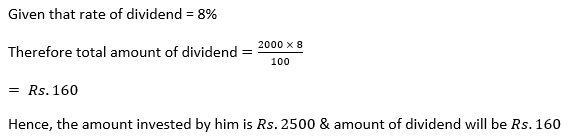4. Find the market price of 5% share when a person gets a dividend of Rs 65 by investing Rs. 1430.
Solution:
Given, amount of dividend = Rs.65
Also given that rate of dividend = 5%5. Salman buys 50 shares of face value Rs 100 available at Rs 132.
(i) what is his investment?
(ii) If the dividend is 7.5% p.a., what will be his annual income?
(iii) If he wants to increase his annual income by Rs 150, how many extra shares should he buy?
Solution:
Given face value = Rs 100
(i) Given that market value (M.V.)= Rs 132
And number of shares = 50
∴  investment = number of shares × market value
= 50 × 132
= Rs 6600
Hence, his investment is Rs.6600
(ii) We have income per share = 7.5% of face value
= (75/ 10 × 100) × 100
= Rs.7.5
∴ annual income = 7.5 × 50
= Rs 375
Hence, his annual income is Rs.375
(iii) Therefore new annual income = 375 + 150 = Rs 525
Number of shares = 525/7.5 = 70
∴ number of extra share to be increased = 70 – 50
= 20
Hence, he buy 20 extra shares.

6. A lady holds 1800,Rs.100 shares of a company that pays 15% dividend annually. Calculate her annual dividend. If she had bought these shares at 40% premium, what percentage return does she get on her investment? Give your answer to the nearest integer.
Solution:
Given total number of shares = 1800
Nominal value of each share = Rs.100
And rate o dividend = 15%
Now, the Total face value of 1800 shares = 100 × 1800
= Rs.180000
∴ total dividend = 180000 × 15/100
= Rs.2700
∴ market value of each share = 100 + 40 = Rs.140
Now the total investment = 140 × 1800
= Rs.252000
∴ percentage on his return
= (27000 × 100)/ 252000
= 10.7%
Hence, she get  11% on her investment.

7. What sum should a person invest in Rs 25 shares, selling at Rs 36, o obtain an income of Rs 720, if the dividend declared is 12%? Also find the percentage return on his income.
(i) The number of shares bought by him.
(ii) The percentage return on his income.
Solution:
Given nominal value of each share = Rs.25
Market value of each share = Rs.36
Total income = Rs 720
Rate of dividend = 12%Total investment = 240 × 36
= Rs 8640
Hence, shares bought by him of Rs. 8640.
(ii) Now, percentage return = (720 × 100)/ 8640
= 8.3%
Hence, 8.3% return on his investment.

8. Ashok invests Rs 26400 on 12% Rs 25 shares of a company. If he receives a dividend of Rs 2475, find:
(i) The number of shares he bought.
(ii) The market value of each share.
Solution:
We have given that,
Ashok invest Rs 26400
Also given Face value of the each share = Rs 25
Rate of dividend = 12%
Total dividend = Rs 2475
We know that, dividend earned = market price of share × number of shares × r/100
(i) Therefore number of shares = (2475/12) × (100/25)
= 825 shares
Hence, 825 shares he bought.
(ii) Market value of each share = (26400/825)
= Rs 32
Hence, the market value (M.V.) of each share is Rs 32

9. Amit kumar invests Rs 36000 in buying Rs 100 shares at Rs 20 premium. The dividend is 15% per annum. Find:
(i) The number of shares he buys
(ii) His yearly dividend
(iii) The percentage return on his investment.
Solution:
We have given that,
Amit Kumar invest for buying shares = Rs 36000
Face value = Rs 100
Premium = Rs 20 and dividend = 15%
(i) Number of shares = 36000/120
= 300 shares
(ii) Dividend = 15% 0f (100 × 300)
= Rs 4500
(iii) Percentage of return = (4500/36000) × 100
= 450/36
= 12.5%
= 13%
Hence, 13% return on his investment.

10. Mr. Tiwari invested Rs 29040 in 15% Rs 100 shares at a premium of 20%. Calculate:
(i) The number of shares bought by Mr. Tiwari
(ii) Mr. Tiwari’s income from the investment
(iii) The percentage return on hid investment.
Solution:
We have given that,
Mr. Tiwari invested Rs.29040
Nominal Value of 1 share Rs 100
Market value of 1 share =Rs.100+20% of Rs.200
(i) Market value of one share = [(200/100) × 100] + 100
= Rs 120
Hence, shares bought by Mr. Tiwari of Rs.120
Number of shares = investment/ market value of one share
=29040/120
= 242
Hence, 242 shares he purchased.
(ii) Income from investment = 242 × 15
= Rs 3630
Hence, his income from the investment is Rs.3630
(iii) Percentage return on his investment = (dividend/ market value) × 100
= (15/120) × 100
= 12.5%
Hence, 12.5% return on his investment.

11. A man buys shares at the par value of Rs yielding 8% dividend at the end of a year. Find the number of shares bought if he receives a dividend of Rs 300.
Solution:
We have given that,
Face value of each share = Rs 10
Rate of dividend = 8% per annum
Total dividend = Rs 300
∴  total face value of shares = (300 × 100)/ 8
= Rs 3750
Number of shares =3750/10
= 375
Hence, he bought 375 shares.

12. A man invests Rs 8800 on buying shares of face value of rupees hundred each at a premium of 10%. If he earns Rs 1200 at the end of year as dividend, find:
(i) The number of shares he has in this company
(ii) The dividend percentage per share.
Solution: We have given that, A man investment = Rs 8800
Face value of each share = Rs 100
Market value of each share = 100 + 10 = Rs 110
Total income = Rs 1200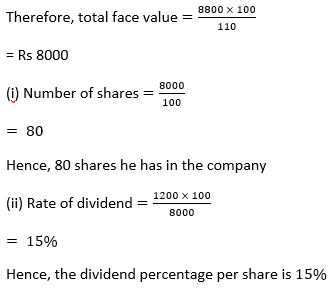13. A man invested Rs 45000 in 15% Rs 100 shares quoted at Rs 125. When the market value of these shares rose to Rs 140, he sold some shares, just enough to raise Rs 8400. Calculate:
(i) The number of shares he still holds.
(ii) The dividend due to him on these shares.
Solution:
We have given that,
A man invested on shares = Rs 45000
Face value of each share = Rs 125
Therefore total number of shares =45000/125
= 360 shares
Income from sold shares = Rs 8400
Therefore number of shares sold = income from shares/ number of shares sold
=8400/140
= 60
(i) Number of shares he still holds = 300
Hence, 300 shares he still hold.
(ii) Market value of 300 shares = 300 × 140
= Rs 42000
Face value of 300 shares = 300 × 125
= Rs 37500
Difference = Market value – face value
= 42000 – 37500
= Rs 4500
Hence, Rs.4500 dividend due to him on these shares.

14. A company pays a dividend of 15% on its ten – rupee shares from which it deducts tax at the rate of 22%. Find the annual income of a man, who owns one thousand shares of his company.
Solution:
We have given that,
Number of shares = 1000
Face value of each share = Rs 10
Rate of dividend = 15%
Rate of tax deducted = 22%
Total face value of 1000 shares = 10 × 1000
= 10000
Total dividend = 10000 × (15/100)
= Rs 1500
Tax deducted at the rate of 22% = 1500 × (22/100)
= Rs 330
Net annual income = 1500 – 330
= 1170
Hence, annual income of a man is Rs.1170

15. Ajay owns 560 shares of a company. The face value of each share is Rs 25. The company declares a dividend 0f 9%. Calculate
(i) The dividend that Ajay will get
(ii) The rate of interest, on his investment if Ajay has paid Rs. 30 for each share.
Solution:
We have given that,
Number of shares = 560
Face value of each share = Rs 25
Rate of dividend = 9% per annum
∴ Total face value of 560 shares = 25 × 560
= Rs 14000
(i) Amount of dividend = 14000 × (9/100)
= Rs 1260
Hence, Ajay will get Rs.1260 dividend.
(ii) Market value of each share = Rs 30
Total investment = 30 × 560
= Rs 1680016. A company with 10000 shares of nominal value of Rs 100 declares an annual dividend of 8% to the share holders.
(i) Calculate the total amount of dividend paid by the company
(ii) Ramesh bought 90 shares of company at Rs 150 per share.
Calculate the dividend he received and the percentage return on his investment.
Solution:
We have given that,
Number of shares = 10000
Nominal value of each share = Rs 100
Rate of annual dividend = 8%
∴ Total face value of 10000 shares = 100 × 10000
= Rs 1000000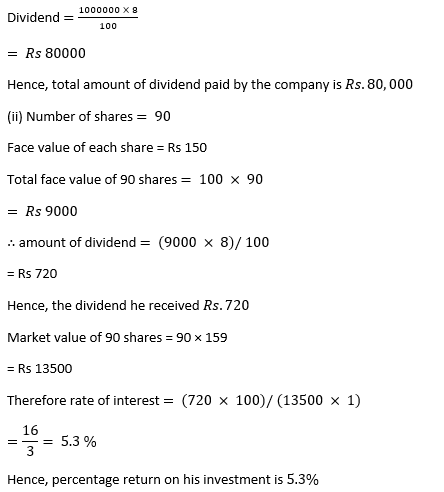17. A company with 4000 shares of nominal value of Rs. 110 declares annual dividend of 15%. Calculate :
(i) the total amount of dividend paid by the company,
(ii) the annual income of Shah Rukh who holds 88 shares in the company,
(iii) if he received only 10% on his investment, find the price Shah Rukh paid for each share. (2008)
Solution:
We have given that,
Number of shares = 4000
Nominal (face) value of each share = Rs.110
Total face value of 4000 shares = Rs.110× 4000
= Rs,440000
Rate of annual dividend = 15%
(i) Amount of dividend = (440000× 15)/ 100
= Rs 66000
Hence, the total amount of dividend paid by the company is Rs.66000
(ii) Number of shares, Shah Rukh has = 88
Face value of 88 shares = 88× 110
= Rs 9680
Annual dividend = (9680×15)/ 100
= Rs 1452
Hence, the total annual income of Shahrukh is Rs.1452
(iii) Rate of annual income on his investment = 10%
∴ His investment = (1452×100)/ 10
= Rs 14520
Market value of each share =14520/88
= Rs 165
Hence, The price Shah Rukh paid for each year (2008) is Rs.165.

18. By investing Rs. 7500 in a company paying 10 percent dividend, an income of Rs. 500 is received. What price is paid for each Rs. 100 share.
Solution:
We have given that,
Investment = Rs. 7500
Rate of dividend = 10%,
∴ Total income = Rs.500.
Now, Face value of each share = Rs.100
∴ Total face value = (100 × 500)/10
= Rs 5000
If face value is Rs.5000, then investment = Rs.7500
and if face value is Rs. 100 then market value of each share = (7500 × 100)/ 5000
= Rs 150
Hence, price is paid for each 100 pair is Rs.150.

19. A man invests Rs. 8000 in a company paying 8% dividend when a share of face value of Rs. 100 is selling at Rs. 60 premium,
(i) What is his annual income,
(ii) What percent does he get on his money ?
Solution:
We have given that,
Investment = Rs. 8000
Face value of each share = Rs. 100
∴ Market value = Rs.100 + Rs.60
= Rs.160
Rate of dividend = 8% p.a.
(i) Annual income = (8× 8000)/160
= Rs 400
Hence, his annual income is Rs.400
(ii) Rate of interest on his money = (400 × 100)/ 8000
= 5%
Hence, 5% he get on his money.

20. A man buys 400 ten-rupee shares at a premium of Rs. 2.50 on each share. If the rate of dividend is 8%, Find,
(i) his investment (ii) dividend received (iii) yield.
Solution:
We have given that,
No. of shares = 400
Face value of each share = Rs. 10
Market value of each share
= Rs.10 + Rs.2.50
= Rs.12.50
Rate of dividend = 8%
Therefore, face value of 400 shares = 10 × 400
= Rs 4000
(i) Total investment = 12.50×400
= Rs 5000
Hence, his investment is Rs.5000
(ii) Total dividend = 4000× (8/100)
= Rs 320
Hence, A man received Rs.320 dividend.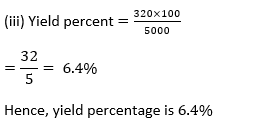21. A man invests Rs. 10400 in 6% shares at Rs. 104 and Rs. 11440 in 10.4% shares at Rs. 143. How much income would he get in all?
Solution: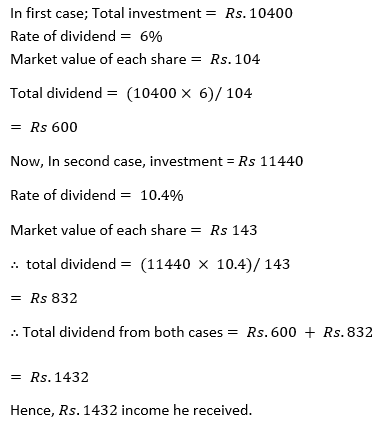22. Two companies have shares of 7% at Rs. 116 and 9% at Rs. 145 respectively. In which of the shares would the investment be more profitable ?
Solution:
Let the investment in each case = Rs.116× 145
For 1st company,
Nominal value =Rs.100
Given, Rate of interest =7%
Given, Market value =Rs.116
Dividend in first case,
= (116× 145× 7)/ 116
= Rs 1015
For 2nd company,
We have given that,
Market value =Rs.145
Now, Dividend in second case
= (116× 145× 9)/ 145
= Rs 1044
∴ To investment in Rs 145 shares is more profitable.

23. Which is better investment: 6% Rs.100 shares at Rs.120 or 8% Rs. 10 shares at Rs.15.
Solution:
Let the investment in each case = Rs.120
In the fist case,
We have given that,
Income on Rs.120 =6% of Rs.100= Rs.6
And,
In second case,
∴ Dividend on Rs. 10
= (8× 10)/ 100
= 0.8
Now,  Income on Rs 15 = 0.8
Then dividend on Rs 120 = (0.8× 120)/ 15
= Rs 6.4
Clearly, 16 > 15
From the above it is clear that, 8% at Rs 10shares at 15 is more profitable.
∴ The investment in the second case is better than the investment in the first case &

24. A man invests Rs -10080 in 6% hundred- rupee shares at Rs.112. Find his annual income. When the shares fall to Rs.96 he sells out the shares and invests the proceeds in 10% ten-rupee shares at Rs.8. Find the change in his annual income.
Solution:
We have given that,
Investment = Rs.10080
Face value of each share = Rs.100
Market value of each share = Rs.112
Rate of dividend = 6%
∴ Total income for the year
= (10080×6)/ 112
= Rs 540
Number of shares =10080/112= 90
∴  Selling price of 90 shares at the rate of Rs 96 each = 90× 96
= Rs 8640
Rate of dividend in new shares = 10%
Face value of each share = Rs 10
Market value of each share = Rs 8
Number of shares = 8640/8 = 1080
Now, Face value of 1080 shares = 1080×10
= Rs 10800
Dividend = (10800× 10)/100
= Rs 1080
∴  Difference in income = 1080 – 540
= Rs 540 more
Hence, the charge in his annual income is Rs.540.

25. A man bought 360 ten-rupee shares paying 12% per annum. He sold them when the price rose to Rs. 21 and invested the proceeds in five-rupee shares paying 4 ½ % per annum at Rs.3.5 per share. Find the annual change in his income.
Solution:
We have given that,
No. of shares bought = 360
Face value of each share = Rs.10
Rate of dividend = 12%
∴  Total face value of 360 shares
= Rs.10 × 360
= Rs.3600
Yearly dividend = (3600×12)/100
= Rs 432
∴  On selling the share at Rs 21, the amount received = 21×360
= Rs 7560
Now, Face value of new shares = Rs 5
Market value = Rs 3.5
Rate of dividend = 4 ½ % = 9/2 %
∴  Number of shares purchased = 7560/3.5
= (7560 ×10)/35
= 2160
Face value of 2160 shares = 5× 2160
= Rs 10800
∴  Dividend = (10800×9)/ (100× 2)
= Rs 486
Change in income = 486 – 432
= Rs 54 gain.
Hence, Rs.54 is the annual change in income.

26. A person invests Rs. 4368 and buys certain hundred-rupee shares at 91. He sells out shares worth Rs. 2400 when they have risen to 95 and the remainder when they have fallen to 85. Find the gain or loss on the total transaction.
Solution:
We have given that,
Investment = Rs.4368
Market value of each share = Rs.91
Face value of each share = Rs 100
∴, number of shares = 4368/91
= 48
And, the Face value of 24 shares = 24× 100
= Rs 2400
Now, the Sale price of shares worth Rs 2400 = (2400×95)/ 100
= Rs 2280
Face value of remaining shares = 24× 100
= Rs 2400
Remainder when they have fallen is Rs.85 [given]
Sale price of shares of remaining amount = (2400× 85)/ 100
= Rs 2040
∴ Total amount received = 2280 + 2040
= Rs 4320
Loss = 4368 – 4320
= Rs 48.
Hence, Rs.48 loss on his total transaction.

27. By purchasing Rs.50 gas shares for Rs.80 each, a man gets 4% profit on his investment. What rate percent is company paying? What is his dividend if he buys 200 shares ?
Solution:
We have given that,
Market value (M.V.) of each share = Rs 80
Face value (F.V.) of each share = Rs.50
The man gets profit on investment = 4%
Dividend on Rs 80 = (80× 4)/ 100
=32/10=3.20
∴ on Rs.50 the company pays Rs.3.20
Percent dividend = 3.20× (100/50)
=320/50= 6.4%
Hence, the rate percent paid by the company is 6.4%
We got income on 1 share is Rs.3.20
Number of shares = 200
∴ income on 200 shares =Rs.3.20×200
Rs.640
= Rs 640
Hence, his dividend if he buys 200 shares is Rs.640.

28. Rs.100 shares of a company are sold at a discount of Rs.20. If the return on the investment is 15%. Find the rate of dividend declared.
Solution:
We have given that,
Market value of each shares = 100 – 20 = Rs.8029. A company declared a dividend of 14%. Find tire market value of Rs.50 shares if the return on the investment was 10%.
Solution:
Rate of dividend = 14%
Dividend on Rs.50 = (14 x 50)/ 100
= Rs 7
Now Rs.10 is interest on the investment of = Rs. 100
Rs.7 will be the interest on = (100 x 7)/ 10 = Rs.70
Hence, Market value of Rs.50 shares = Rs.70

30. At what price should a 6.25% Rs. 100 shares be quoted when the money is worth 5%?
Solution:
We have given that,
Interest on Rs.100 worth = Rs.5
If interest is Rs.5, then market value = Rs.100
and if interest is Rs.6.25, then market value
Now, use the formula,
Dividend ⇒ d × F.V. × n (no. of shares)
Worth = dividend / investment × 100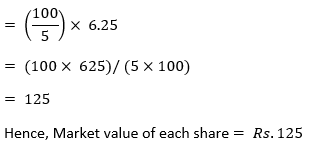Chapter Test

1. If a man received ₹1080 as dividend from 9% ₹20 shares, find the number of shares purchased by him.
Solution:
We have given that,
Dividend rate =9%
Face value =Rs.20
Income on one share = 9/100 ×20
= Rs 9/5
Therefore, no. of shares = 1080×5/9
= 120× 5= 600
Hence, 600 shares purchased by him.

2. Find the percentage interest on capital invested in 18% shares when a Rs 10 share costs Rs 12.
Solution:
We have given that,
Dividend rate =18%
Face value =Rs.10
Dividend on one share = 18% of Rs 10
= (18× 10)/100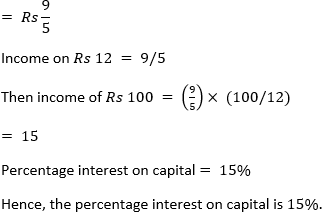3. Rohit Kulkami invests Rs 10000 in 10% Rs 100 shares of a company. If his annual dividend is Rs 800, find :
(i) The market value of each share.
(ii) The rate percent which he earns on his investment.
Solution:
We have given that,
Investment = Rs 10000
Face value of each share = Rs 100
Rate of dividend = 10%
Annual dividend = Rs 800
(i) Market value = (10000 × 10)/ 800
= Rs 125
Hence, the market value of each share is Rs.125
(ii) rate percent on investment = (800 × 100)/ 10000
= 8%
Hence, the rate percent is 8% he earn on his investment.

4. At what price should a 9% Rs 100 share be quoted when the money is worth 6% ?
Solution:
We have given that,
If interest is 6 then investment = Rs 100
and if interest is 9%, then investment
= Rs (100× 9)/ 6
= Rs 150
Hence , Market value of each share = Rs 150

5. By selling at Rs 92, some 2.5% Rs 100 shares and investing the proceeds in 5% Rs 100 shares at Rs 115, a person increased his annual income by Rs 90. Find:
(i) the number of shares sold.
(ii) the number of shares purchased.
(iii) the new income.
(iv) the rate percent which he earns on his investment.
Solution:
As we have given that,
Rate of dividend = 2.5%
& market price = Rs 92
(i) Let number of shares purchased = x
Selling price of x shares = 92 x
Income from investing
Rs x = (92x× 2.5)/ 92
= (92x× 25)/ (92× 10) = (5/2) x